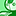# QlikView App Dev

Discussion Board for collaboration related to QlikView App Development.

Announcements
Join us at the Cloud Data and Analytics Tour! REGISTER TODAY
cancel
Showing results for
Search instead for
Did you mean:Partner

## help combining variables

Hi all,

i have the following variables..

vCounter = Only({<nd_FISCAL_PERIOD = {\$(=Only(CurrentFiscalPeriod))}>}Counter)

vPeriod_1 = Only({<Counter = {\$(=vCounter-1)}>} nd_FISCAL_PERIOD)

as you can see, vPeriod_1 uses vCounter within it.

i need to combine the above 2 variables, the vCounter expression needs to replace the vCounter part of the vPeriod_1 variable expression.

then the i want to replace the vPeriod_1 part of the below expression with the combined expression from the above.

='("LV Snapshot FY\$(=Right(CurrentFiscalYear,2))*" & (*\$(vPeriod_1)))'

i cant get this to work, can anyone help please?

i have attached an example qvw.

thanks

1 Solution

Accepted SolutionsMVP

Maybe like

='LV Snapshot FY\$(=Right(CurrentFiscalYear,2)) \$(=Only({<Counter = {\$(=Only({<nd_FISCAL_PERIOD = {\$(=Only(CurrentFiscalPeriod))}>}Counter)-1)}>} nd_FISCAL_PERIOD)),LV Snapshot FY\$(=Right(CurrentFiscalYear,2)) \$(=Only({<Counter = {\$(=Only({<nd_FISCAL_PERIOD = {\$(=Only(CurrentFiscalPeriod))}>}Counter)-2)}>} nd_FISCAL_PERIOD)),LV Snapshot FY\$(=Right(CurrentFiscalYear,2)) \$(=Only({<Counter = {\$(=Only({<nd_FISCAL_PERIOD = {\$(=Only(CurrentFiscalPeriod))}>}Counter)-3)}>} nd_FISCAL_PERIOD))'

15 RepliesMVP

Maybe

='("LV Snapshot FY\$(=Right(CurrentFiscalYear,2))*" & (*\$(=Only({<Counter = {\$(=Only({<nd_FISCAL_PERIOD = {\$(=Only(CurrentFiscalPeriod))}>}Counter)-1)}>} nd_FISCAL_PERIOD)))'Partner
Author

Hi Stefan,

thanks again! that works in QV (i changed the button to use that expression instead of the variable) but again it doesnt work in NP! AAARRRRRGGGGHHHHHH!!!!!

this is so frustrating!MVP

Does it work if you hard code the search string in NP?

("LV Snapshot FY17*" & (*201704)

Just to check that the search approach does work out.Partner
Author

ok so the above doesnt work, it only works if the actual string is hardcoded

i.e.

LV Snapshot FY17 201704

so the problem is getting NP to evaluate the expression and apply the result.MVP

Sorry, I missed a bracket:

("LV Snapshot FY17*" & (*201704))

or

("LV Snapshot FY17*" & "*201704")Partner
Author

i had already noticed that and corrected it in my test. didnt work.

within a text box, the expression...

='("LV Snapshot FY\$(=Right(CurrentFiscalYear,2))*" & (*\$(=Only({<Counter = {\$(=Only({<nd_FISCAL_PERIOD = {\$(=Only(CurrentFiscalPeriod))}>}Counter)-1)}>} nd_FISCAL_PERIOD))))'

gives the result of..

("LV Snapshot FY17*" & (*201704))

but this isnt a value in the Dataset field which i think is why its not making the selection.Partner
Author

i have changed the expression slightly to..

='LV Snapshot FY\$(=Right(CurrentFiscalYear,2)) \$(=Only({<Counter = {\$(=Only({<nd_FISCAL_PERIOD = {\$(=Only(CurrentFiscalPeriod))}>}Counter)-1)}>} nd_FISCAL_PERIOD))'

which removed some double quotes and some brackets, this in a text box matches one of the values in the Dataset field and also works in NP.

i dont know if this will have any other affect though so i will add in the other 2 periods and see what happensMVP

Where are you using this search string in the context of NP?

Above is a compound search string, which should work in selection -select in field triggers, for example.

Compound Search - demystifiedPartner
Author

in NP i am creating a field filter on the dataset field and ticking the 'evaluate' check box (i have also tried without ticking the check box too.